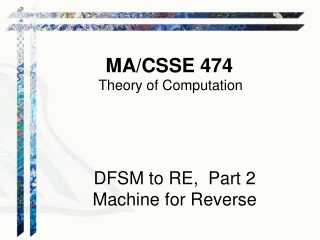DownloadDownload PresentationMA/CSSE 474

# MA/CSSE 474

Download Presentation## MA/CSSE 474

- - - - - - - - - - - - - - - - - - - - - - - - - - - E N D - - - - - - - - - - - - - - - - - - - - - - - - - - -
##### Presentation Transcript

1. MA/CSSE 474 Theory of Computation DFSM to RE, Part 2 Machine for Reverse

2. Your Questions? • HW 6 or 7 problems • Exam 1 questions • Anything else • Previous class days' material • Reading Assignments

3. Recap: Kleene’s Theorem Finite state machines and regular expressions define the same class of languages. To prove this, we must show: Theorem: Any language that can be defined by a regular expression can be accepted by some FSM and so is regular. Done Days 11-12. Theorem: Every regular language (i.e., every language that can be accepted by some DFSM) can be defined with a regular expression. We will review what we did Day 12, and finish the algorithm and example today.

4. For Every FSM There is a Corresponding Regular Expression • We’ll show this by construction. The construction is different than the textbook's. • Let M = ({q1, …, qn}, , , q1, A) be a DFSM.Define Rijk to be the set of all strings x  * such that • (qi,x) |-M (qj, ), and • if (qi,y) |-M (q𝓁, ), for any prefix y of x (except y=  and y=x), then 𝓁  k • That is, Rijk is the set of all strings that take us from qi to qj without passing through any intermediate states numbered higher than k. • In this case, "passing through" means both entering and immediately leaving. • Note that either i or j (or both) may be greater than k. * *

5. Example: Rijk • Rijk is the set of all strings that take us from qi to qj without passing through any intermediate states numbered higher than k. • In this case, "passing through" means both entering and leaving. • Note that either i or j (or both) may be greater than k. R110 R111 R112 R131 R132 R330 R333 R142 R143

6. DFSMReg. Exp. construction • Rijk is the set of all strings that take M from qi to qj without passing through any intermediate states numbered higher than k. • Examples: Rij0 Rijn • We will show that for all i,j{1, …, n} and all k {0, …, n}, Rijk there is a regular expression rijk that defines Rijk. • Also note that L(M) is the union of R1jn over all qj in A. • We know that the union of languages defined by reg. exps. is defined by a reg. exp.

7. DFSMReg. Exp. continued • Rijk is the set of all strings that take M from qi to qj without passing through any intermediate states numbered higher than k. It can be computed recursively: • Base cases (k = 0): • If i  j, Rij0 = {a : (qi, a) = qj} • If i = j, Rii0 = {a : (qi, a) = qi}  {} • Recursive case (k > 0): Rijk is Rij(k-1)  Rik(k-1)(Rkk(k-1))*Rkj(k-1) • We show by induction that each Rijk is defined by some regular expression rijk.

8. DFSMReg. Exp. Proof pt. 1 • Base case definition (k = 0): • If i  j, Rij0 = {a : (qi, a) = qj} • If i = j, Rii0 = {a : (qi, a) = qi}  {} • Base case proof:Rij0 is a finite set of symbols, each of which is either  or a single symbol from . So Rij0 can be defined by the reg. exp. rij0 = a1a2…ap (or a1a2…ap if i=j),where {a1, a2, …,ap} is {a  : (qi, a) = qj. • Note that if M has no direct transitions from qi to qj, then rij0 is  (it is  if i=j and no "loop" on that state).

9. DFSMReg. Exp. Proof pt. 2 • Recursive definition (k > 0): Rijk is Rij(k-1)  Rik(k-1)(Rkk(k-1))*Rkj(k-1) • Induction hypothesis: For each 𝓁 and 𝓂, there is a regular expression r𝓁𝓂(k-1) such that L(r𝓁𝓂(k-1) )= R𝓁𝓂(k-1). • Induction step. By the recursive parts of the definition of regular expressions and the languages they define, and by the above recursive definition of Rijk :Rijk = L(rij(k-1)  rik(k-1)(rkk(k-1))*rkj(k-1))

10. DFAReg. Exp. Proof pt. 3 • We showed by induction that each Rijk is defined by some regular expression rijk. • In particular, for all qjA, there is a regular expression r1jn that defines R1jn. • Then L(M) = L(r1j1n … r1jpn ), where A = {qj1, …, qjp}

11. An Example (rijk is rij(k-1)  rik(k-1)(rkk(k-1))*rkj(k-1)) Start q1 q2 q3 0 1 0 0,1 1 k=0 k=1 k=2 r11k   (00)* r12k 00 0(00)* r13k 1 1 0*1 r21k 0 0 0(00)* r22k    00 (00)* r23k 1 1  01 0*1 r31k   (0  1)(00)*0 r32k 0  1 0  1 (0  1)(00)* r33k    (0  1)0*1

12. Another "machine construction" example Given a DFSM M=(Κ, Σ, δ, s, A) that accepts a language L(M), construct a FSM M' such that L(M') = L(M)R • Write a careful mathematical description of that machine • Prove that L(M') = L(M)R